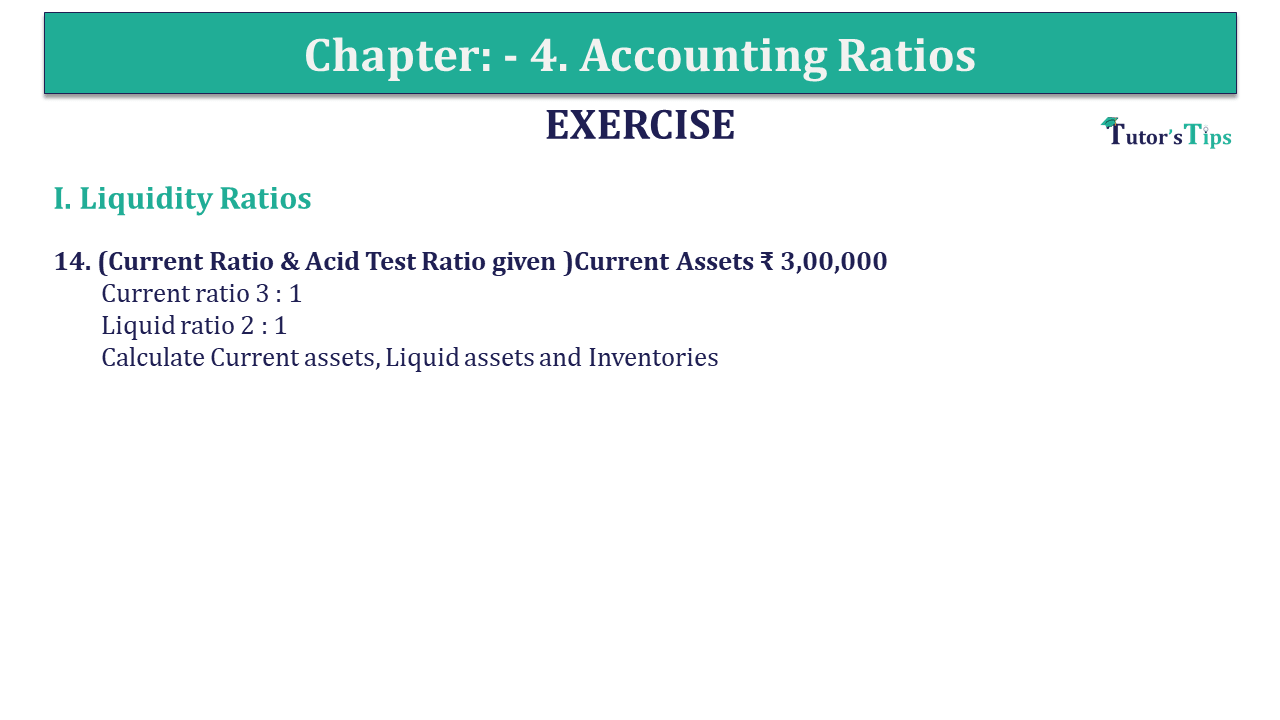# Question 14 Chapter 4 of +2-B – USHA Publication 12 ClassQ-14- CH-4 Book 2 - Usha Pub. +2 Book 2020 - Solution

Question 14 Chapter 4 of +2-B

I. Liquidity Ratios

14. (Current Ratio & Acid Test Ratio given )Current Assets ₹ 3,00,000
Current ratio 3 : 1
Liquid ratio 2 : 1
Calculate Current assets, Liquid assets and Inventories

## The solution of Question 14 Chapter 4 of +2-B: –

Solution: – (a)

 Current Ratio = 3 : 1 Current Liabilities = 3,00,000 Current Assets = ₹ 3,00,000 3 = ₹ 1,00,000

Solution: – (b)

 Liquid Ratio = 2 : 1 Current Liabilities = ₹ 1,00,000 (Calculated above) Quick Assets = Current Liabilities x 2 = ₹ 1,00,000 x 2 = ₹ 2,00,000

Solution: – (c)

 Inventories = Current Assets – Quick Assets = ₹ 3,00,000 – ₹ 2,00,000 = ₹ 1,00,000

What are Liquidity Ratios – Formulas and Examples

Comment if you have any question.

Also, Check out the solved question of previous Chapters: –